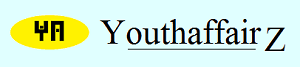November 2014

# Solution

The length of the track has not been mentioned. Let it be "x" kilometres. I complete two rounds in one hour; one round is "x" kilometres, so two rounds mean 2x kilometres. Thus, my speed is 2x kilometres per hour. Similarly, my friend's speed is 3x kilometres per hour.

When we walk in the opposite direction, the relative speed is the sum of my friend's speed and my speed (please check the October 2013 teaser, "Going round in circles"). However, when we walk in the same direction, the relative speed is the difference between my friend's speed and my speed. The rest is the same.

Therefore, in this case, the relative speed = 3x - 2x = x kilometres per hour.

The distance to be covered is "x" kilometres (the length of the track).

Now, speed = distance/time.

Therefore x = x/t.

Therefore, t = x/x, or t = one hour.

Thus, we will pass each other after one hour. Thereafter, we will pass each other again after covering subsequent "x" kilometres. This means we will pass each other for the second time after 2x kilometres, the third time after 3x kilometres, fourth time after 4x kilometres, and so on.

Thus, for the second meeting, distance = 2x and speed = x. Time = distance/speed, so t = 2x/x = 2 hours. Likewise, for the third meeting, t = 3 hours, we meet for the fourth time after 4 hours, and so on.

However, we have walked only for one-and-half hours. We are, therefore, interested only in what occurs during these 90 minutes and not beyond. During these 90 minutes, we have passed each other only once (the second meeting would have occurred after two hours). So, my answer is: we pass each other only once. Did I get that right?

• Union Public Service Commission - www.upsc.gov.in
• IIT-Kharagpur - www.iitkgp.ac.in
• Indian Statistical Institute - www.isical.ac.in
• Indian Institute of Technology Madras - www.iitm.ac.in
• Indian Institute of Management, Ahmedabad - www.iimahd.ernet.in
• Indian Institute of Mass Commission - www.iimc.nic.in
• IIT Bombay - www.iitb.ac.in